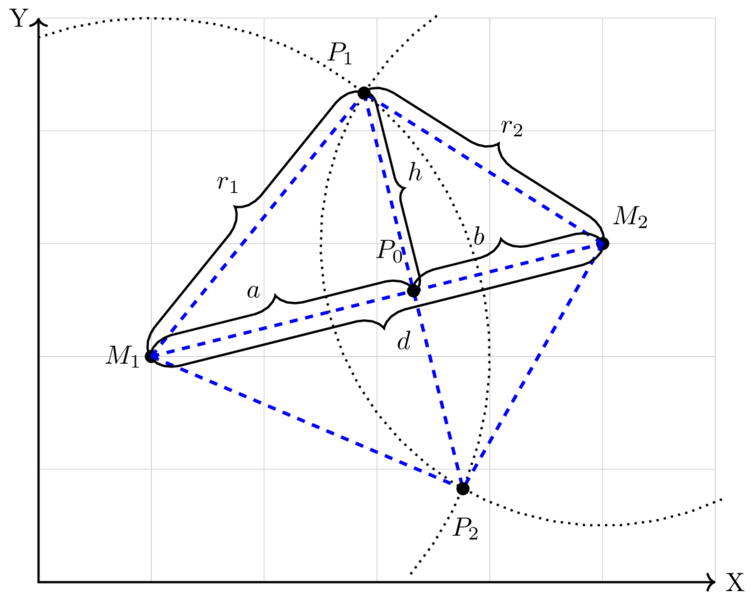# Intersections of Circles

Date 2014-08-09

In my education as a computer scientist, I once got an assignment where I had to calculate all pairwise intersections of N circles. I spent three hours finding out how to calculate the intersections between two circles, because that was not sufficiently explained anywhere. I intend to solve and explain the solution to this problem because I find it important to not only know how something works, but also why it works.

### Subject

The question I intend to answer is the following:

Given the coordinates of the centres of two circles and their ranges, how do we compute the intersections?

I will also show why my solution is correct after I present it.

### Required knowledge

• Basic vector calculus

• Basic geometry

### Naming

• Suppose we have two circles $C_1$ and $C_2$.

• Centres of the circles: $M_1$ and $M_2$.

• Intersection points: $P_1$ and $P_2$.

• The coordinates of M_1 and M_2: $(x_1,y_1)$ and $(x_2,y_2)$ respectively.

• The distance between $M_1$ and $M_2$: $d$.

• The radii of the circles: $r_1$ and $r_2$ respectively.

• The line between $M_1$ and $M_2$: $l_1$.

• The line between $P_1$ and $P_2$: $l_2$.

• The intersection of $l_1$ and $l_2$: $P_0$.

• The distance from $M_1$ to $P_0$: $a$.

• The distance from $M_2$ to $P_0$: $b$.

• The distance from $P_0$ to $P_1$: $h$.

• The distance from $P_0$ to $P_2$: $h'$.

an illustration will clarify this:### How to calculate whether two circles intersect

1. $d \leftarrow \Vert M_2-M_1 \Vert$

2. return $(d \le r_1 + r_2 ) \wedge (d \ge |r_1 − r_2|)$

This is a very short way of saying: "If the circles are close enough to each other, they intersect"

### How to calculate the circles' intersections

This an algorithm to calculate the intersections of two circles.

1. If $C_1$ = $C_2$ then return $C_1$

2. If $C_1$ and $C_2$ don't intersect then return $\emptyset$

3. $d \leftarrow \Vert M_2-M_1 \Vert$

4. $a \leftarrow \frac{r_1^2-r_2^2+d^2}{2d}$

5. $x_0 \leftarrow x_1 + \frac{a}{d}(x_2-x_1)$

6. $y_0 \leftarrow y_1 + \frac{a}{d}(y_2-y_1)$

7. $h \leftarrow \sqrt{r_{1}^{2} - a^{2}}$

8. $x_1' \leftarrow x_0 + \frac{h}{d}(y_2-y_1)$

9. $y_1' \leftarrow y_0 - \frac{h}{d}(x_2-x_1)$

10. If $a = r_1$ then return $\{(x_1',y_1')\}$

11. $x_2' \leftarrow x_0 - \frac{h}{d}(y_2-y_1)$

12. $y_2' \leftarrow y_0 + \frac{h}{d}(x_2-x_1)$

13. return $\{(x_1',y_1'),(x_2',y_2')\}$

For the explanation of why this is true, read on.

### $l_1$ and $l_2$ are perpendicular and $h$ and $h'$ are equal.

The triangles $M_1P_1P_0$ and $M_2P_2P_0$ are equilateral triangles, precisely because $P_1$ and $P_2$ are on the circles. An equilateral triangle can be split into two congruent right triangles. In this case, $M_1P_1P_0$ can be split into $M_1P_1P_0$ and $M_1P_2P_0$ while $M_2P_2P_0$ can be split into $M_2P_1P_0$ and $M_2P_2P_0$.

• Because any of those triangles is a right triangle, $l_1$ and $l_2$ are perpendicular.

• Because $M_1P_1P_0$ and $M_1P_2P_0$ are congruent triangles, $h$ and $h'$ are equal.

### The algorithm is correct.

There are three cases to prove in the algorithm.

• If the circles are not close enough, they don't intersect

• If the circles touch, they have two equal intersection points

• Otherwise, there are two different intersection points

I will only prove the third case. The second case is a special case of the third. The first is trivial.

We now know that the triangle $M_1P_1P_0$ is a right triangle. We can therefore calculate $h$ with Pythagoras.

$$\left{ \begin{array}{c l r} r_1^2 = a^2 + h^2 & (1)\ r_2^2 = b^2 + h^2 & (2) \end{array} \right.$$

Out of the first equation, we can get the value of $h$.

$$h \leftarrow \sqrt{r_1^2-a^2}$$

If we subtract equation two from equation one we get the following equation:

$$\begin{array}{r l} r_1^2 -r_2^2 & = a^2 - b^2\ & = (a-b)(a+b)\ & = d(a-b)\ & = d(a-d+a)\ & = d(2a-d) \end{array}$$

From this equation, we can get the value of $a$.

$$a = \frac{r_1^2-r_2^2 + d^2}{2d}$$

Now we can get the coordinates $(x_0,y_0)$ of $P_0$ because $P_0$ is located on $l_1$ at a distance of $a$ from $M_1$.

$$P_0 = M_1 + \frac{a}{d}(M_2-M_1) = M_2 + \frac{b}{d}(M_1-M_2)$$

# $$\begin{bmatrix} P_{0x}\P_{0y}\ \end{bmatrix} # \begin{bmatrix} x_1 + \frac{a}{d}(x_2-x_1)\ y_1 + \frac{a}{d}(y_2-y_1)\ \end{bmatrix} \begin{bmatrix} x_2 + \frac{a}{d}(x_1-x_2)\ y_2 + \frac{a}{d}(y_1-y_2)\ \end{bmatrix}$$

At last, we can calculate the coordinates of the intersection points from the coordinates of $M_1$, $M_2$ and $P_0$.

# $$\begin{bmatrix} P_x\P_y\ \end{bmatrix} \begin{bmatrix} x_0 \pm \frac{h}{d}(y_2-y_1)\ y_0 \mp \frac{h}{d}(x_2-x_1) \end{bmatrix}$$

### Our assignment, solution and report repository (dutch)

Repository: https://github.com/NorfairKing/TMI-project-2014

Note that you can't use any of our code for school/college/university assignments. Apart from that, refer to the license in the repository to see what you can and can't do with our code.

Obsessed is just a word the lazy use to describe the dedicated. - Russel Warren

Know a technical team that could use strong technical leadership?

The magic of getting up early